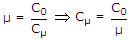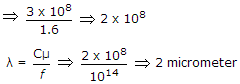# Electronics and Communication Engineering - Electromagnetic Field Theory - Discussion

3.

Refractive index of glass is 1.5. Find the wavelength of a beam of light with a frequency of 1014 Hz in glass. Assume velocity of light is 3 x 108 m/sec in vacuum.

 [A]. 4 μm [B]. 3 μm [C]. 2 μm [D]. 1 μm

Explanation:

Given, μ = 1.6, f = 1014 Hz, v = 3 x 108 m/sec.Prachi said: (Oct 28, 2015) We can not consider the value of 1.6?

 Bittu said: (Aug 24, 2016) Here refractive index given -1.5. Then why 1.6 considered?

 Shree said: (Aug 7, 2017) Refractive index is mentioned as 1.5 not 1.6. It will get another answer not 2 micrometre.

 Suraj Rathod said: (Sep 10, 2017) Answer is 1.5 um.

 Sunni said: (Sep 14, 2017) No, answer is 2 that is print mistake 1.5 not 1.6.

 Pradeep Rathod said: (Jan 12, 2020) C/f = 3x10^8/10^14 = 3um/1.5 = 2μm.